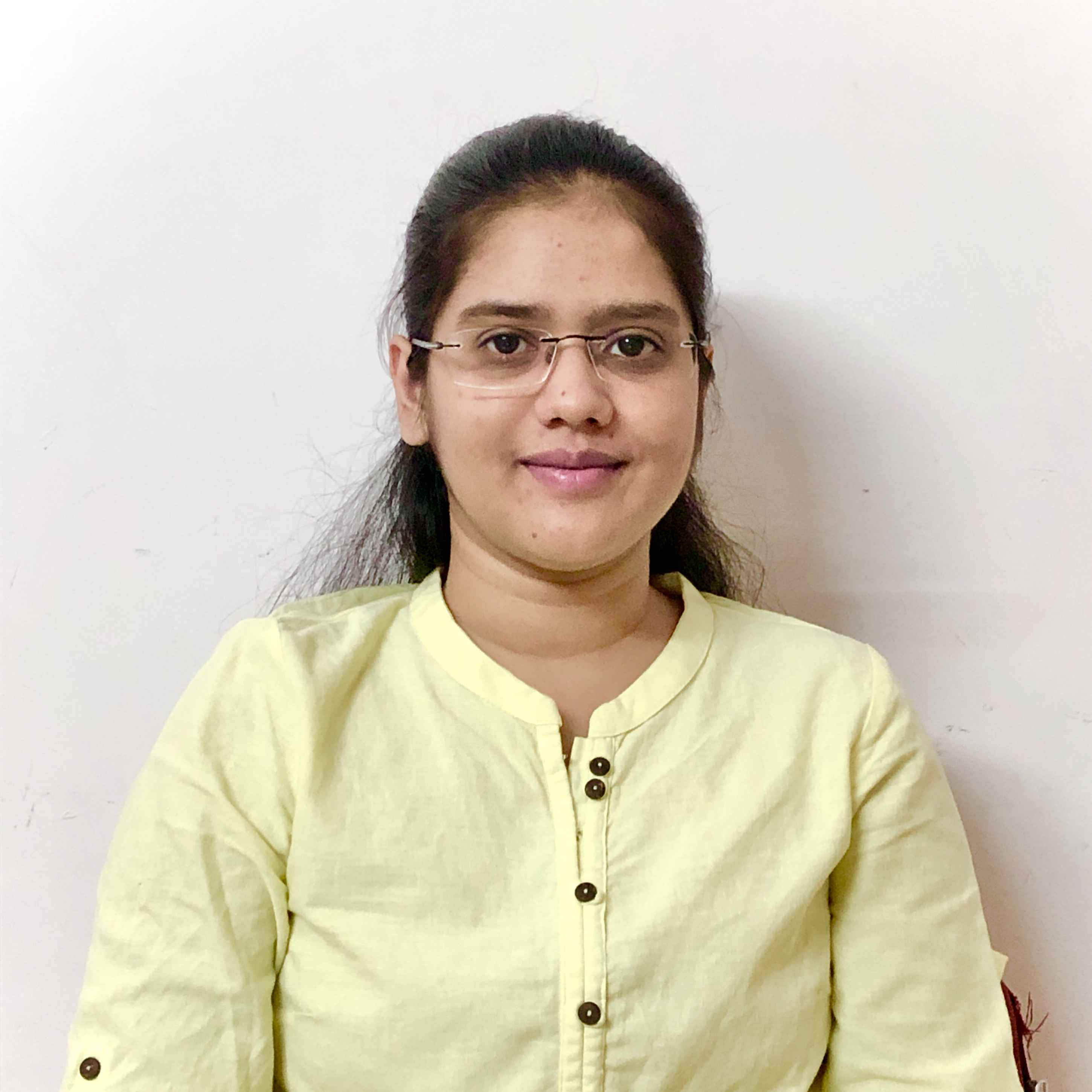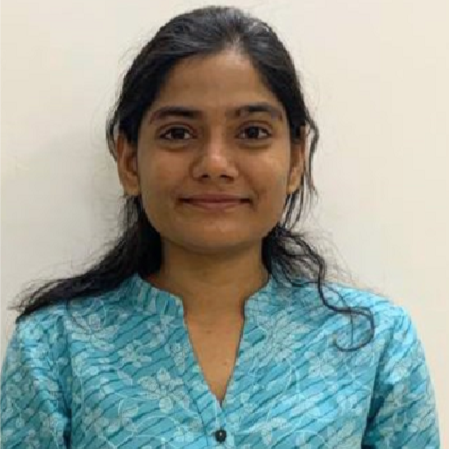X
Conflict free feedback vertex set: A parameterized dichotomy
A. Agrawal, D. Lokshtanov, S. Saurabh
Published in Schloss Dagstuhl- Leibniz-Zentrum fur Informatik GmbH, Dagstuhl Publishing
2018
Volume: 117

Abstract
In this paper we study recently introduced conflict version of the classical Feedback Vertex Set (FVS) problem. For a family of graphs F, we consider the problem F-CF-Feedback Vertex Set (F-CF-FVS, for short). The F-CF-FVS problem takes as an input a graph G, a graph H ∈ F (where V (G) = V (H)), and an integer k, and the objective is to decide if there is a set S ⊆ V (G) of size at most k such that G − S is a forest and S is an independent set in H. Observe that if we instantiate F to be the family of edgeless graphs then we get the classical FVS problem. Jain, Kanesh, and Misra [CSR 2018] showed that in contrast to FVS, F-CF-FVS is W-hard on general graphs and admits an FPT algorithm if F is the family of d-degenerate graphs. In this paper, we relate F-CF-FVS to the Independent Set problem on special classes of graphs, and obtain a complete dichotomy result on the Parameterized Complexity of the problem F-CF-FVS, when F is a hereditary graph family. In particular, we show that F-CF-FVS is FPT parameterized by the solution size if and only if F+Cluster IS is FPT parameterized by the solution size. Here, F+Cluster IS is the Independent Set problem in the (edge) union of a graph G ∈ F and a cluster graph H (G and H are explicitly given). Next, we exploit this characterization to obtain new FPT results as well as intractability results for F-CF-FVS. In particular, we give an FPT algorithm for F+Cluster IS when F is the family of Ki,j-free graphs. We show that for the family of bipartite graph B, B-CF-FVS is W-hard, when parameterized by the solution size. Finally, we consider, for each 0 < < 1, the family of graphs F, which comprise of graphs G such that |E(G)| ≤ |V (G)|2−, and show that F-CF-FVS is W-hard, when parameterized by the solution size, for every 0 < < 1. © Akanksha Agrawal, Pallavi Jain, Lawqueen Kanesh, Daniel Lokshtanov, and Saket Saurabh.
••